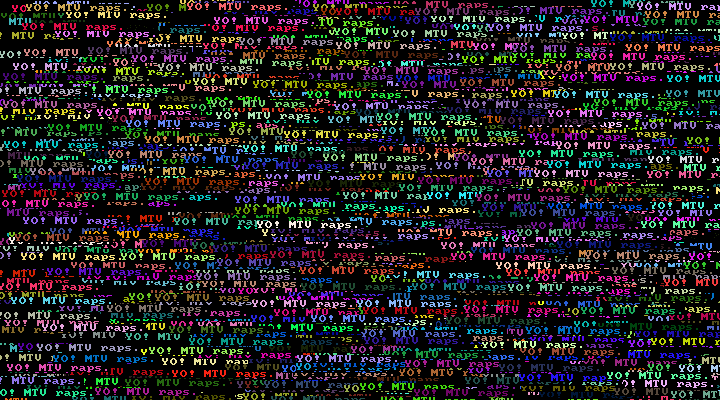# Hardware Agnostic Graphics Library for EmbeddedWhat started as a project to learn C, I have now been using in all my hobby projects. HAGL is a hardware agnostic graphics library for embedded projects. It supports basic geometric primitives, bitmaps, blitting, fixed width fonts and an optional framebuffer.

## Yet another graphics library?

Most embedded hobbyist projects do not seem to care about code reuse. Instead there is a graphics library for every different display driver for every different architecture. They all implement the same functions for drawing the graphical primitives This feels wrong. Graphics library should not know anything about the underlying hardware, responsibilities should be separated.

HAGL takes a different approach. It contains code only for drawing the primitives. It can be used with any microcontroller and display driver. It can also be used with normal computers. This is useful for testing your graphics code without need to flash it to the microcontroller.

## Hardware abstraction layer

To use Copepod with your microcontroller and display driver you must provide a HAL. The only mandatory function for HAL to provide is for putting a single pixel on the display. Copepod will use the putpixel funtion to draw all the other graphical primitives. For examples of this see libgd HAL and libsdl2 HAL.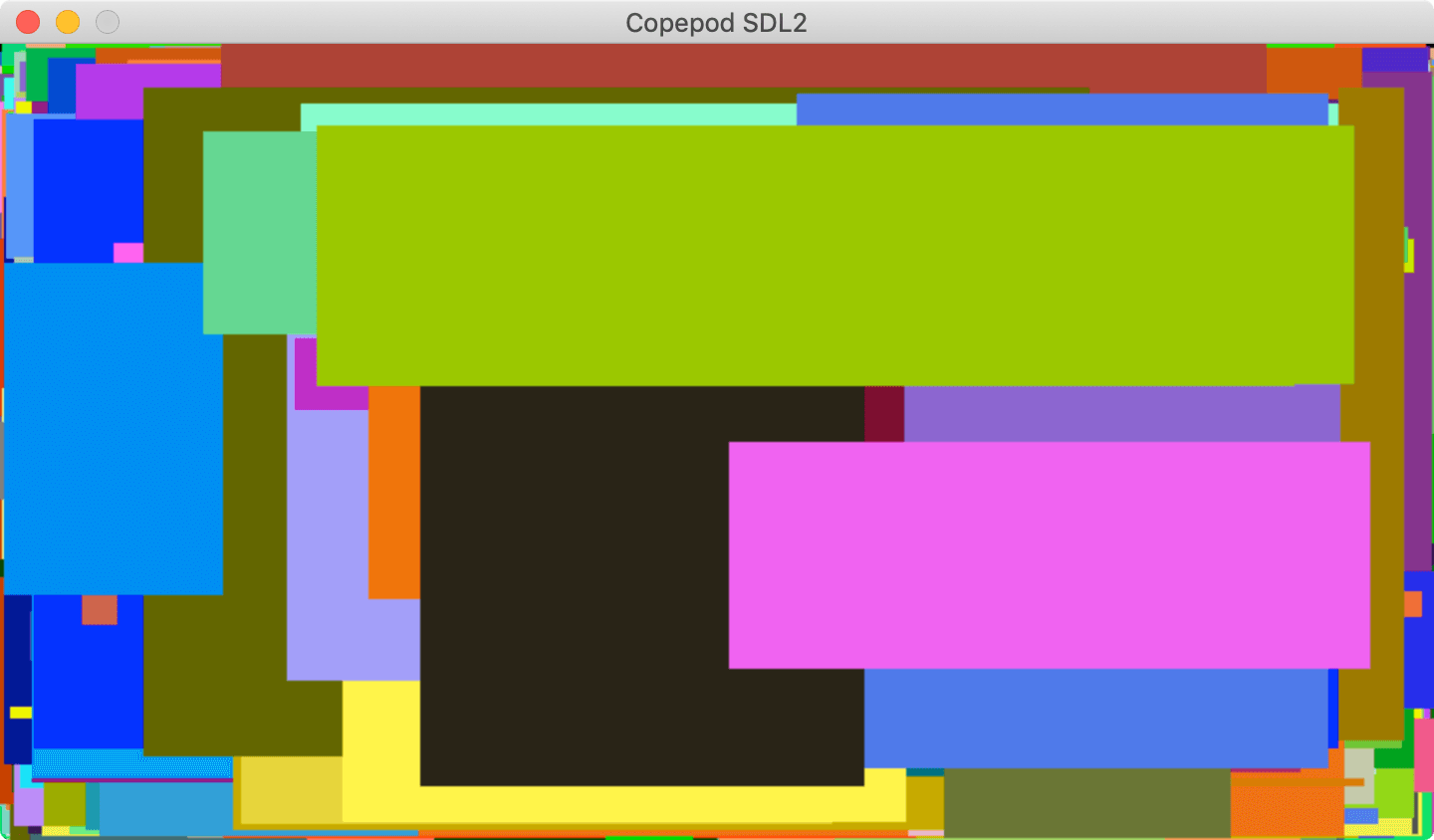For improved speed the HAL can also provide accelerated functions for bitmap blitting and horizontal and vertical lines. See ESP MIPI DCS HAL for an example of a hardware accelerated HAL. This is also the one I use with my ESP32 projects. It supports most of the displays hobbyists currently use.

## How fast is it?

Speed mostly depends on two things. Everything is much faster when double buffering is enabled. Also the HAL implementation dictates a lot. I have been testing with the TTGO T-Display, TTGO T4, M5StickC and M5Stack.

In the below table numbers are operations per second with double buffering. ESP32 is clocked at the default 160MHz. Bigger number is better. T-Display and M5StickC have higher numbers because they have smaller resolution. Smaller resolution means less bytes to push to the display.

T4 T-Display M5Stack M5StickC
hagl_put_pixel() 304400 304585 340850 317094
hagl_draw_line() 10485 14942 12145 31293
hagl_draw_circle() 15784 16430 17730 18928
hagl_fill_circle() 8712 9344 9982 13910
hagl_draw_ellipse() 8187 8642 9168 10019
hagl_fill_ellipse() 3132 3457 3605 5590
hagl_draw_triangle() 3581 5137 4160 11186
hagl_fill_triangle() 1246 1993 1654 6119
hagl_draw_rectangle() 22759 30174 26910 64259
hagl_fill_rectangle() 2191 4849 2487 16146
hagl_draw_rounded_rectangle() 17660 21993 20736 39102
hagl_fill_rounded_rectangle() 2059 4446 2313 13270
hagl_draw_polygon() 2155 3096 2494 6763
hagl_fill_polygon() 692 1081 938 3295
hagl_put_char() 29457 29131 32429 27569
hagl_flush() 32 76 32 96

When double buffering is disabled everything is much slower. On the positive side you save lots of memory.

T4 T-Display M5Stack M5StickC
hagl_put_pixel() 16041 15252 16044 24067
hagl_draw_line() 113 172 112 289
hagl_draw_circle() 148 173 145 230
hagl_fill_circle() 264 278 261 341
hagl_draw_ellipse() 84 103 85 179
hagl_fill_ellipse() 114 128 116 191
hagl_draw_triangle() 37 54 37 114
hagl_fill_triangle() 72 111 72 371
hagl_draw_rectangle() 2378 2481 2374 3482
hagl_fill_rectangle() 91 146 91 454
hagl_draw_rounded_rectangle() 458 535 459 808
hagl_fill_rounded_rectangle() 87 139 79 400
hagl_draw_polygon() 21 33 19 71
hagl_fill_polygon() 43 66 49 228
hagl_put_char) 4957 4264 4440 2474
hagl_flush() x x x x

You can run the speed tests yourself by checking out the speedtest repository.

## Graphical functions

The function calls themselves should be pretty self explanatory. Most of them take coordinates and a RGB565 color. Out of bounds coordinates are clipped to the current display.

### Put a pixel

``````for (uint32_t i = 1; i < 100000; i++) {
int16_t x0 = rand() % DISPLAY_WIDTH;
int16_t y0 = rand() % DISPLAY_HEIGHT;
color_t color = rand() % 0xffff;

hagl_put_pixel(x0, y0, color);
}
``````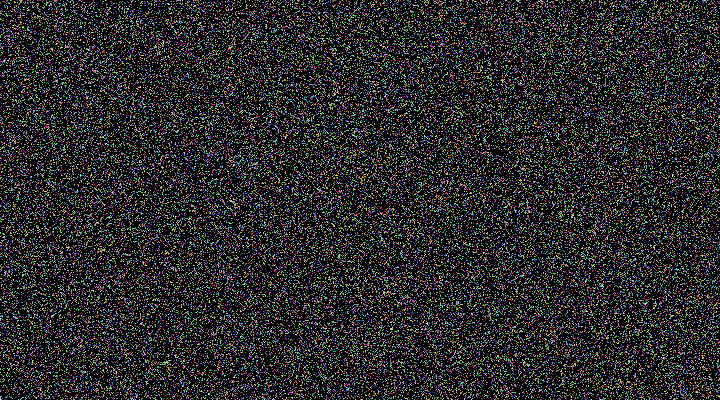### Draw a line

``````for (uint16_t i = 1; i < 1000; i++) {
int16_t x0 = rand() % DISPLAY_WIDTH;
int16_t y0 = rand() % DISPLAY_HEIGHT;
int16_t x1 = rand() % DISPLAY_WIDTH;
int16_t y1 = rand() % DISPLAY_HEIGHT;
color_t color = rand() % 0xffff;

hagl_draw_line(x0, y0, x1, y1, color);
}
``````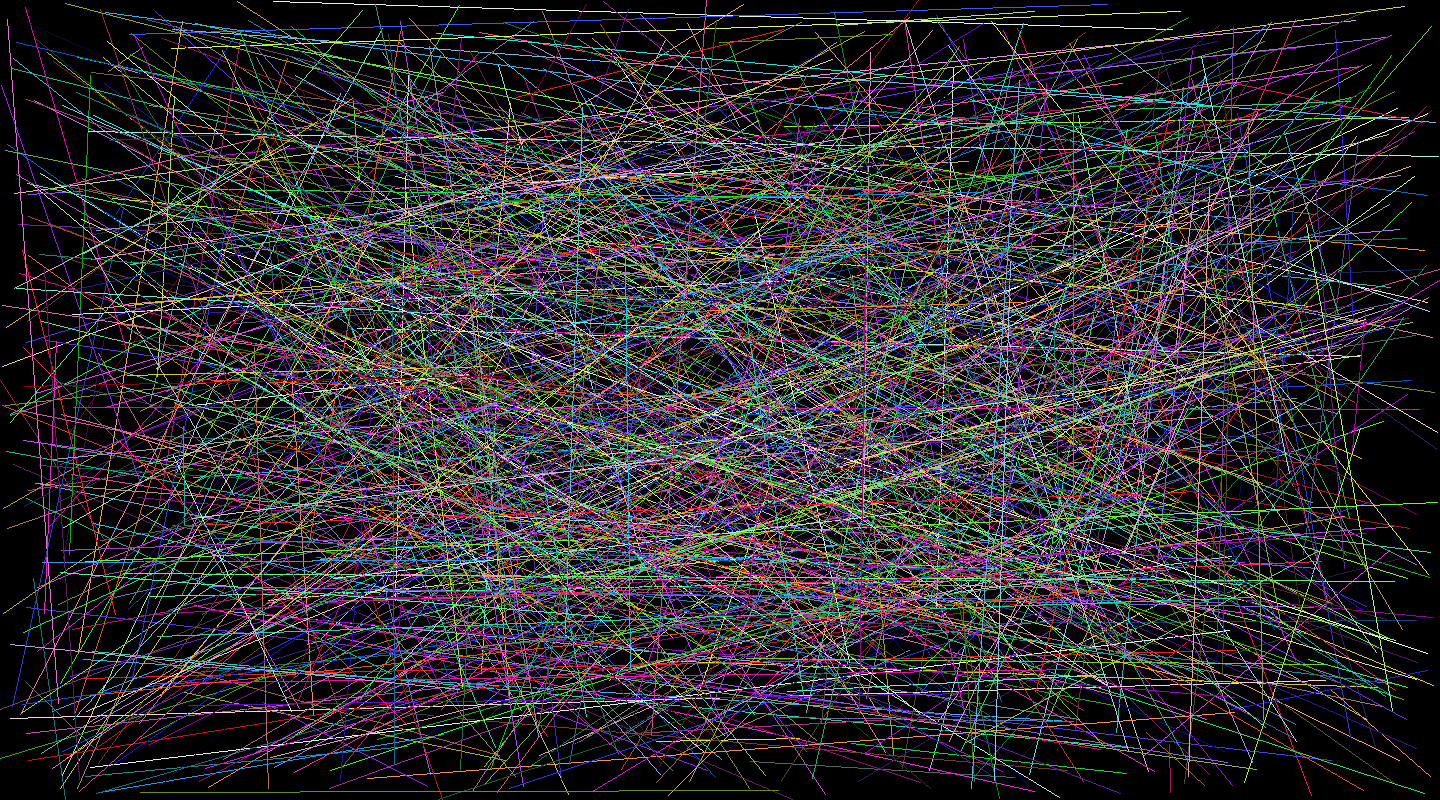### Draw a horizontal line

``````for (uint16_t i = 1; i < 1000; i++) {
int16_t x0 = rand() % (DISPLAY_WIDTH / 2);
int16_t y0 = rand() % DISPLAY_HEIGHT;
int16_t width = rand() % (DISPLAY_WIDTH - x0);
color_t color = rand() % 0xffff;

hagl_draw_hline(x0, y0, width, color);
}
``````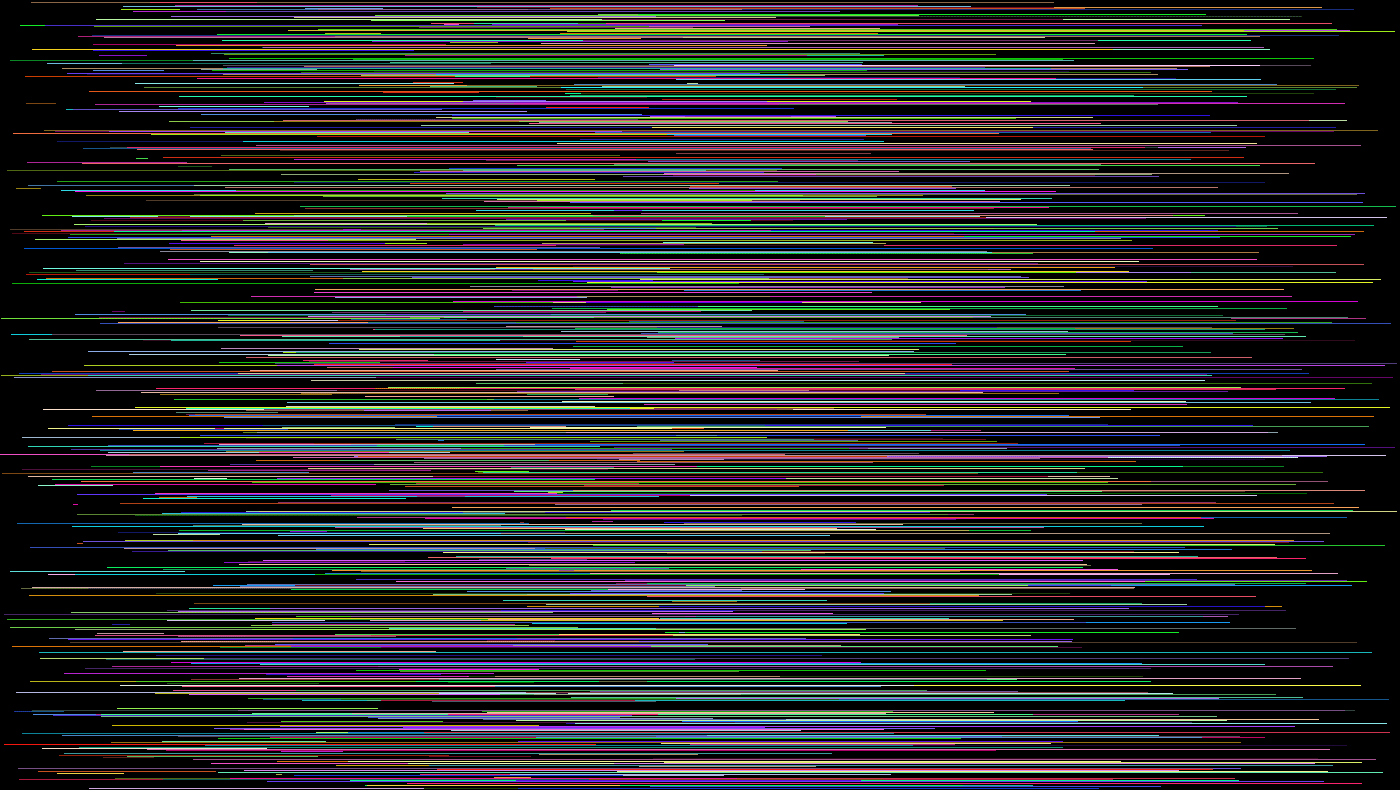### Draw a vertical line

``````for (uint16_t i = 1; i < 1000; i++) {
int16_t x0 = rand() % DISPLAY_WIDTH;
int16_t y0 = rand() % (DISPLAY_HEIGHT / 2);
int16_t height = rand() % (DISPLAY_HEIGHT - y0);
color_t color = rand() % 0xffff;

hagl_draw_vline(x0, y0, height, color);
}
``````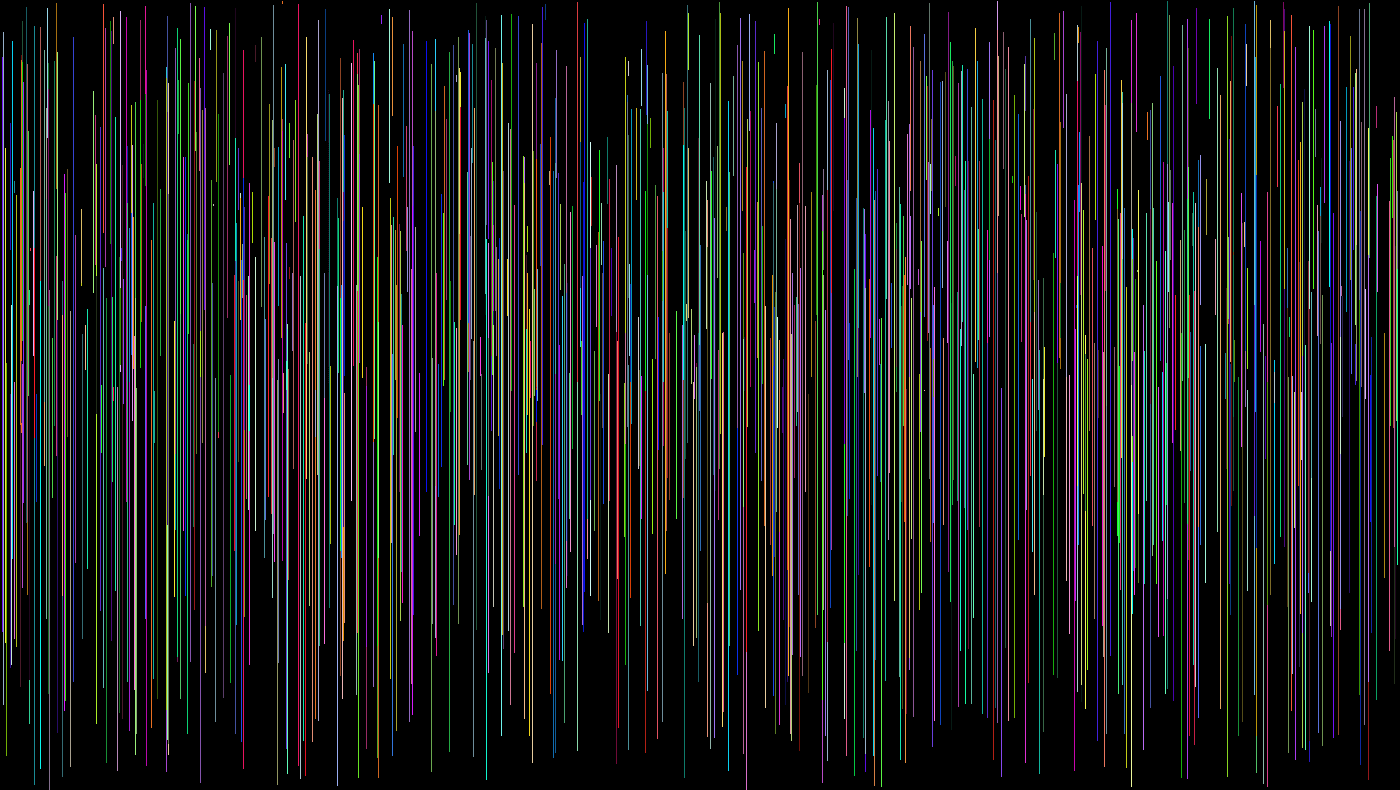### Draw a circle

``````for (uint16_t i = 1; i < 500; i++) {
int16_t x0 = DISPLAY_WIDTH / 2;
int16_t y0 = DISPLAY_HEIGHT / 2;
int16_t radius = rand() % DISPLAY_WIDTH;
color_t color = rand() % 0xffff;

}
``````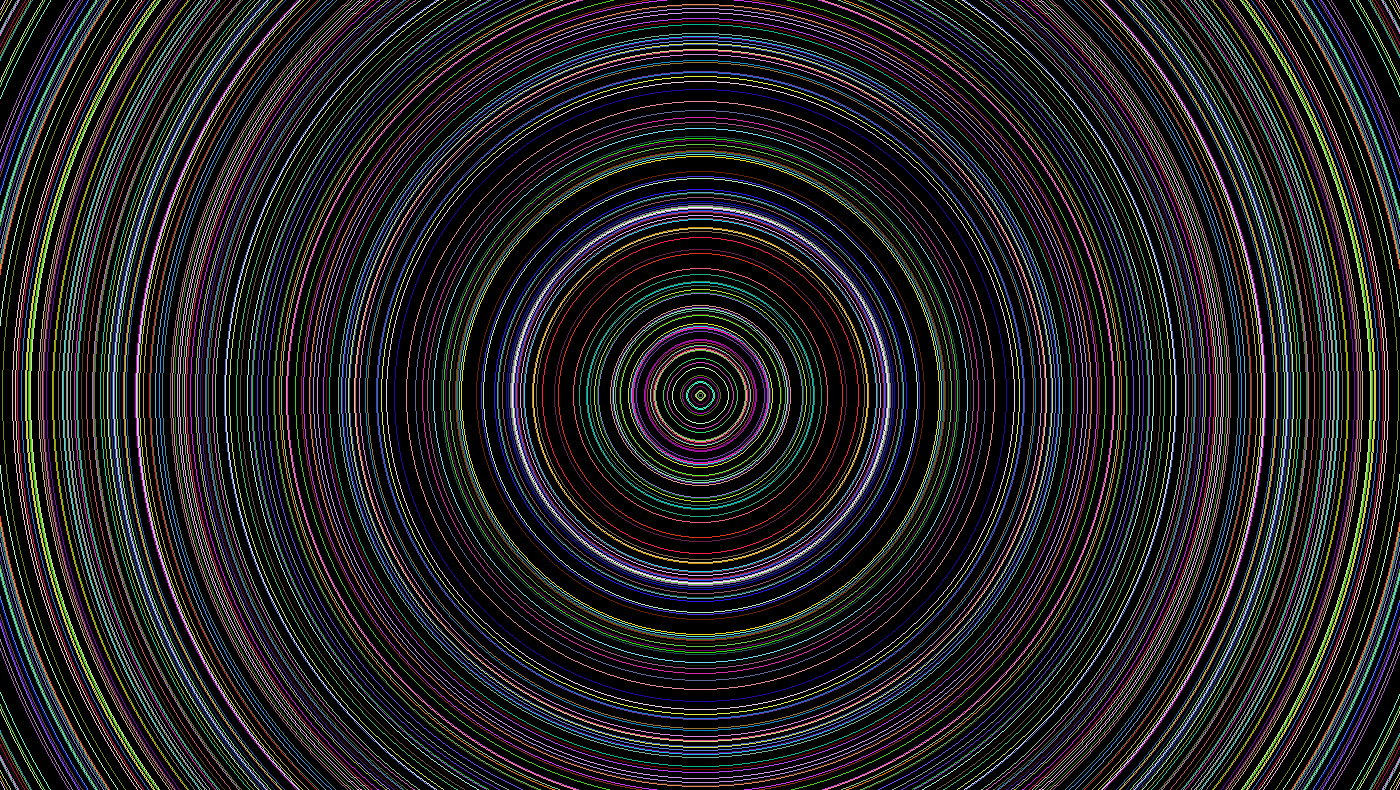### Draw a filled circle

``````for (uint16_t i = 1; i < 500; i++) {
int16_t x0 = rand() % DISPLAY_WIDTH;
int16_t y0 = rand() % DISPLAY_HEIGHT;
int16_t radius = rand() % 100;
color_t color = rand() % 0xffff;

}
``````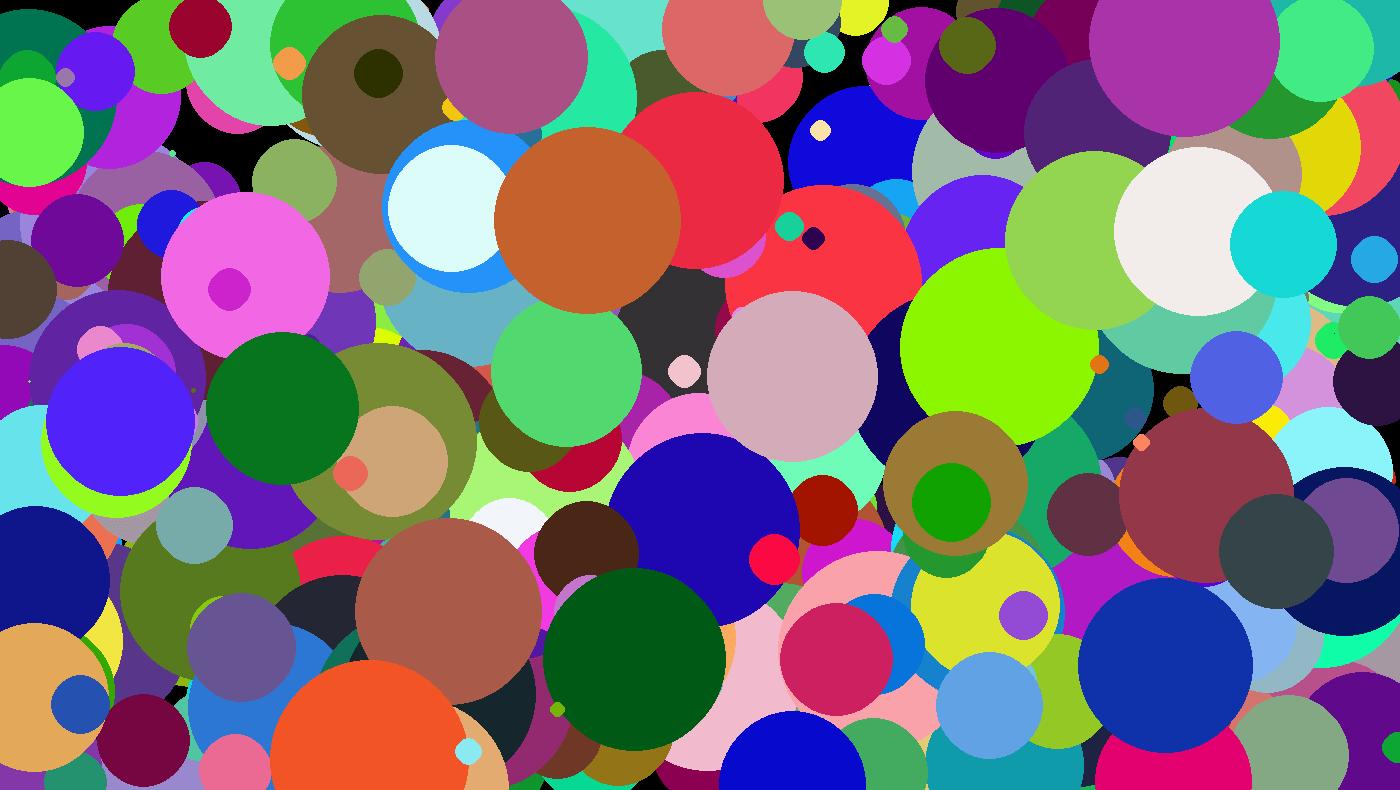### Draw an ellipse

``````for (uint16_t i = 1; i < 500; i++) {
int16_t x0 = DISPLAY_WIDTH / 2;
int16_t y0 = DISPLAY_HEIGHT / 2;
int16_t rx = rand() % DISPLAY_WIDTH;
int16_t ry = rand() % DISPLAY_HEIGHT;
color_t color = rand() % 0xffff;

hagl_draw_ellipse(x0, y0, rx, ry, color);
}
``````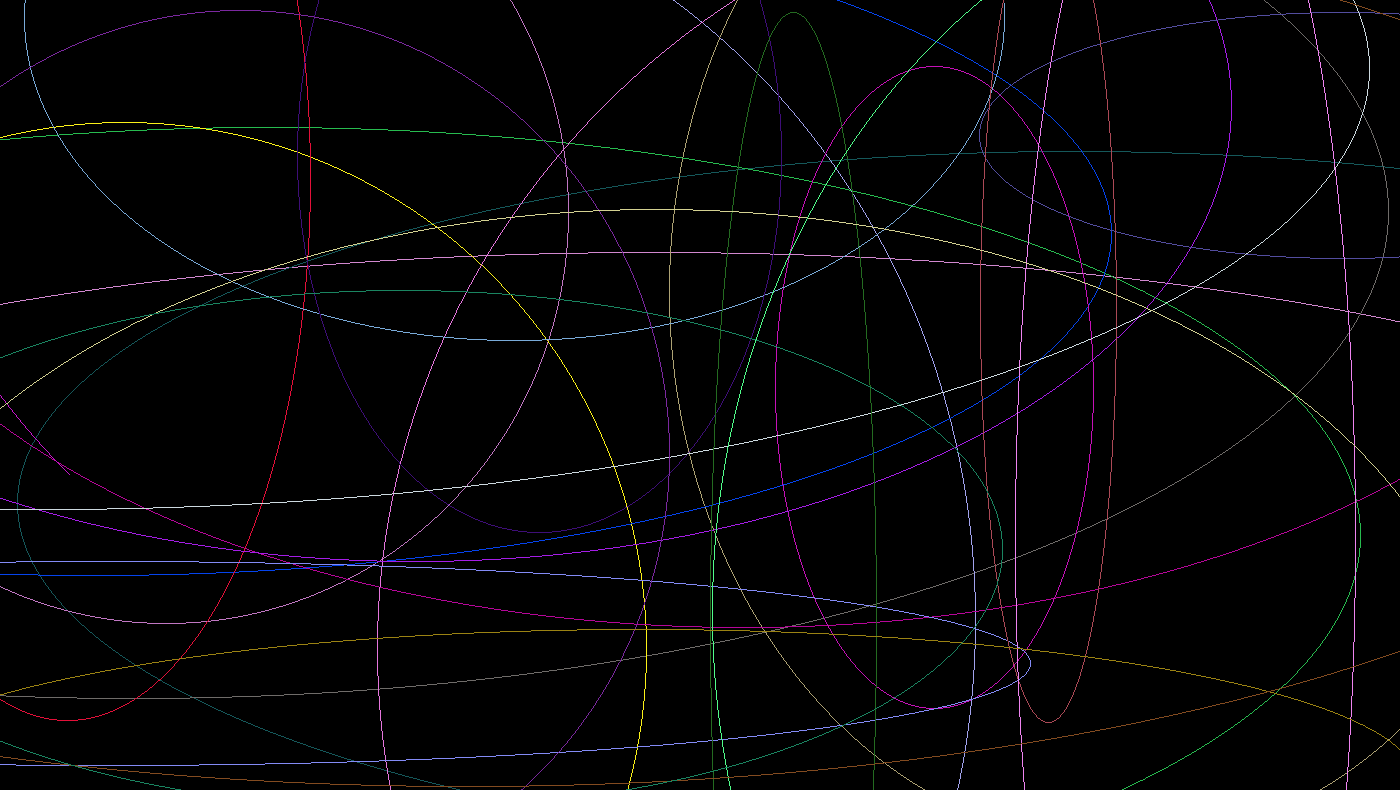### Draw a filled ellipse

``````for (uint16_t i = 1; i < 500; i++) {
int16_t x0 = rand() % DISPLAY_WIDTH;
int16_t y0 = rand() % DISPLAY_HEIGHT;
int16_t rx = rand() % DISPLAY_WIDTH / 4;
int16_t ry = rand() % DISPLAY_HEIGHT / 4;
color_t color = rand() % 0xffff;

hagl_draw_ellipse(x0, y0, rx, ry, color);
}
``````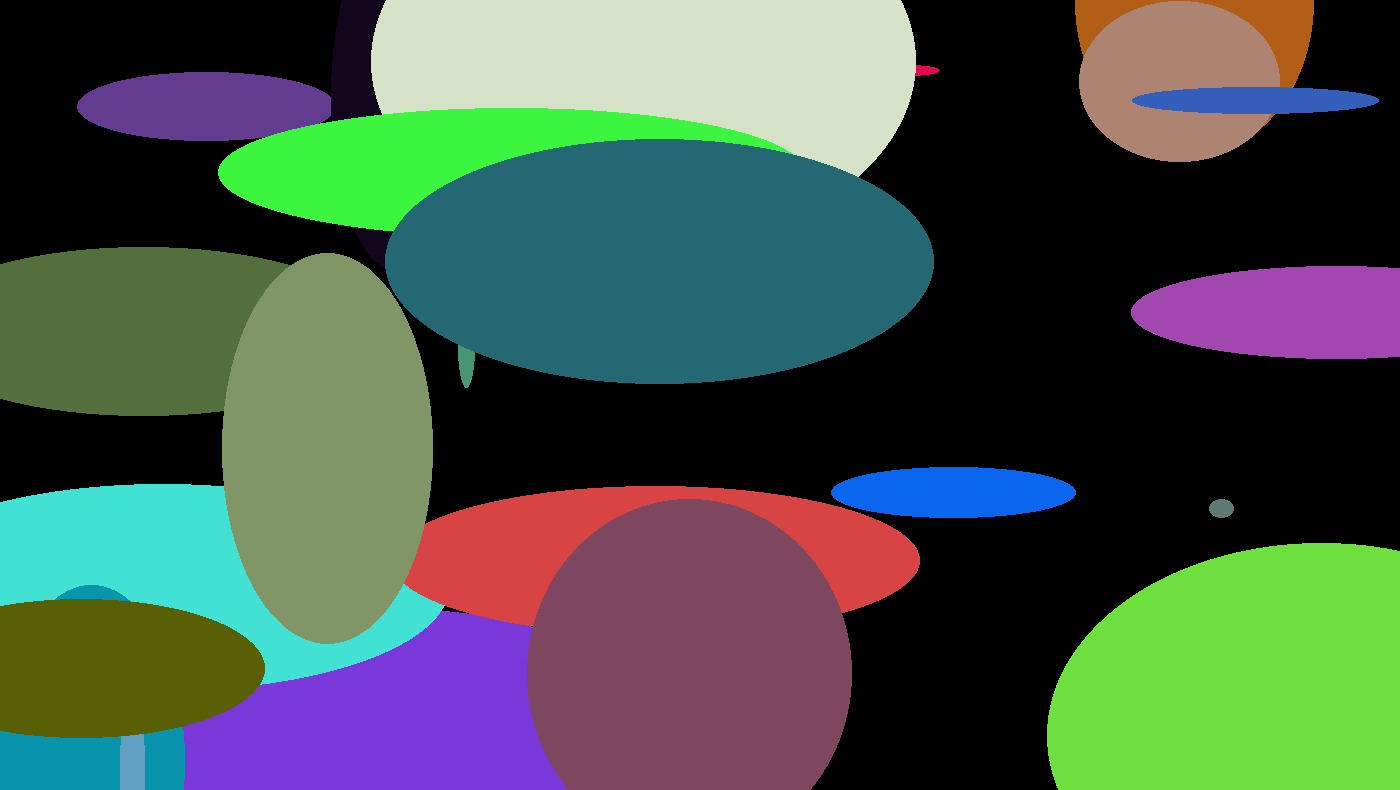### Draw a triangle

``````int16_t x0 = rand() % DISPLAY_WIDTH;
int16_t y0 = rand() % DISPLAY_HEIGHT;
int16_t x1 = rand() % DISPLAY_WIDTH;
int16_t y1 = rand() % DISPLAY_HEIGHT;
int16_t x2 = rand() % DISPLAY_WIDTH;
int16_t y2 = rand() % DISPLAY_HEIGHT;
color_t color = rand() % 0xffff;

hagl_draw_triangle(x0, y0, x1, y1, x2, y2, color);
``````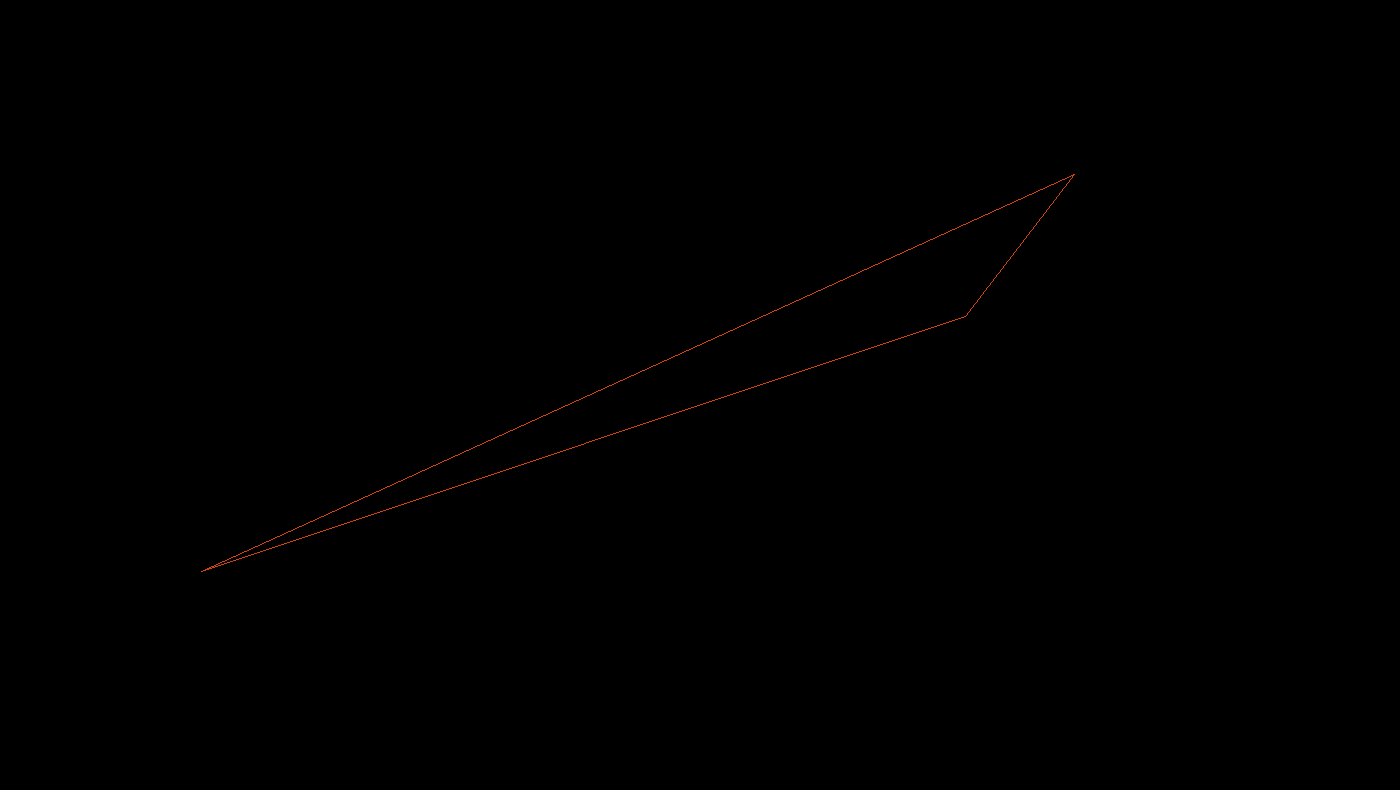### Draw a filled triangle

``````int16_t x0 = rand() % DISPLAY_WIDTH;
int16_t y0 = rand() % DISPLAY_HEIGHT;
int16_t x1 = rand() % DISPLAY_WIDTH;
int16_t y1 = rand() % DISPLAY_HEIGHT;
int16_t x2 = rand() % DISPLAY_WIDTH;
int16_t y2 = rand() % DISPLAY_HEIGHT;
color_t color = rand() % 0xffff;

hagl_fill_triangle(x0, y0, x1, y1, x2, y2, color);
``````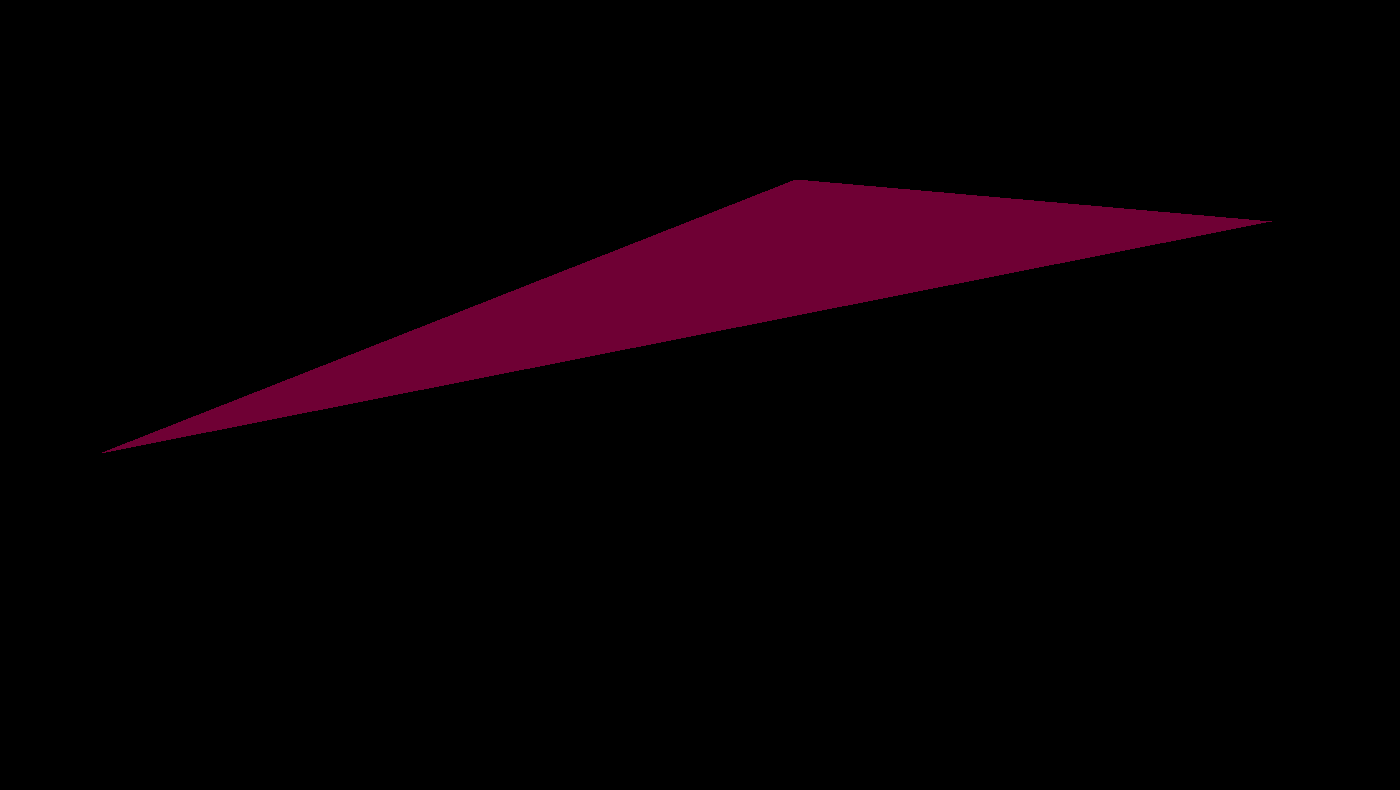### Draw a rectangle

``````for (uint16_t i = 1; i < 50; i++) {
int16_t x0 = rand() % DISPLAY_WIDTH;
int16_t y0 = rand() % DISPLAY_HEIGHT;
int16_t x1 = rand() % DISPLAY_WIDTH;
int16_t y1 = rand() % DISPLAY_HEIGHT;
color_t color = rand() % 0xffff;

hagl_draw_rectangle(x0, y0, x1, y1, color);
}
``````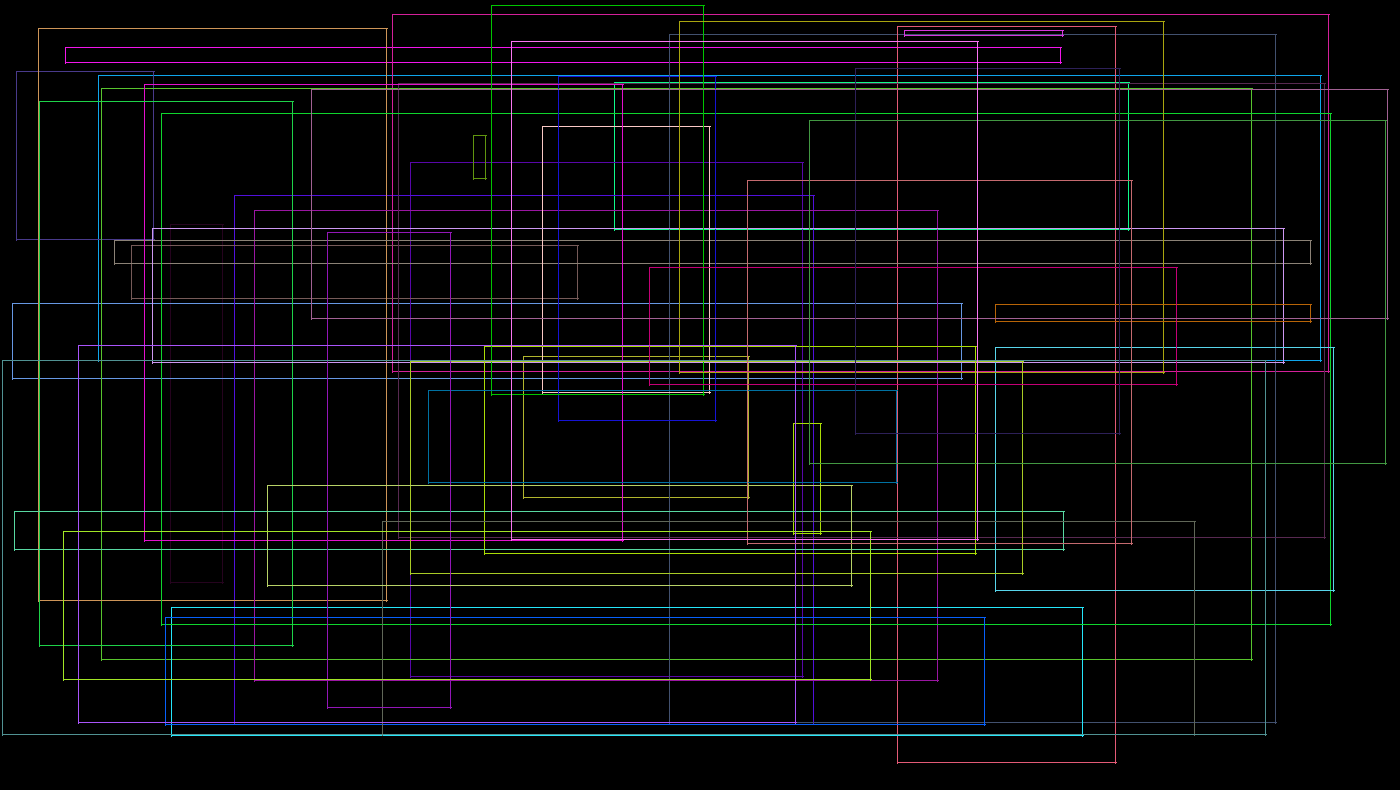### Draw a filled rectangle

``````for (uint16_t i = 1; i < 10; i++) {
int16_t x0 = rand() % DISPLAY_WIDTH;
int16_t y0 = rand() % DISPLAY_HEIGHT;
int16_t x1 = rand() % DISPLAY_WIDTH;
int16_t y1 = rand() % DISPLAY_HEIGHT;
color_t color = rand() % 0xffff;

hagl_fill_rectangle(x0, y0, x1, y1, color);
}
``````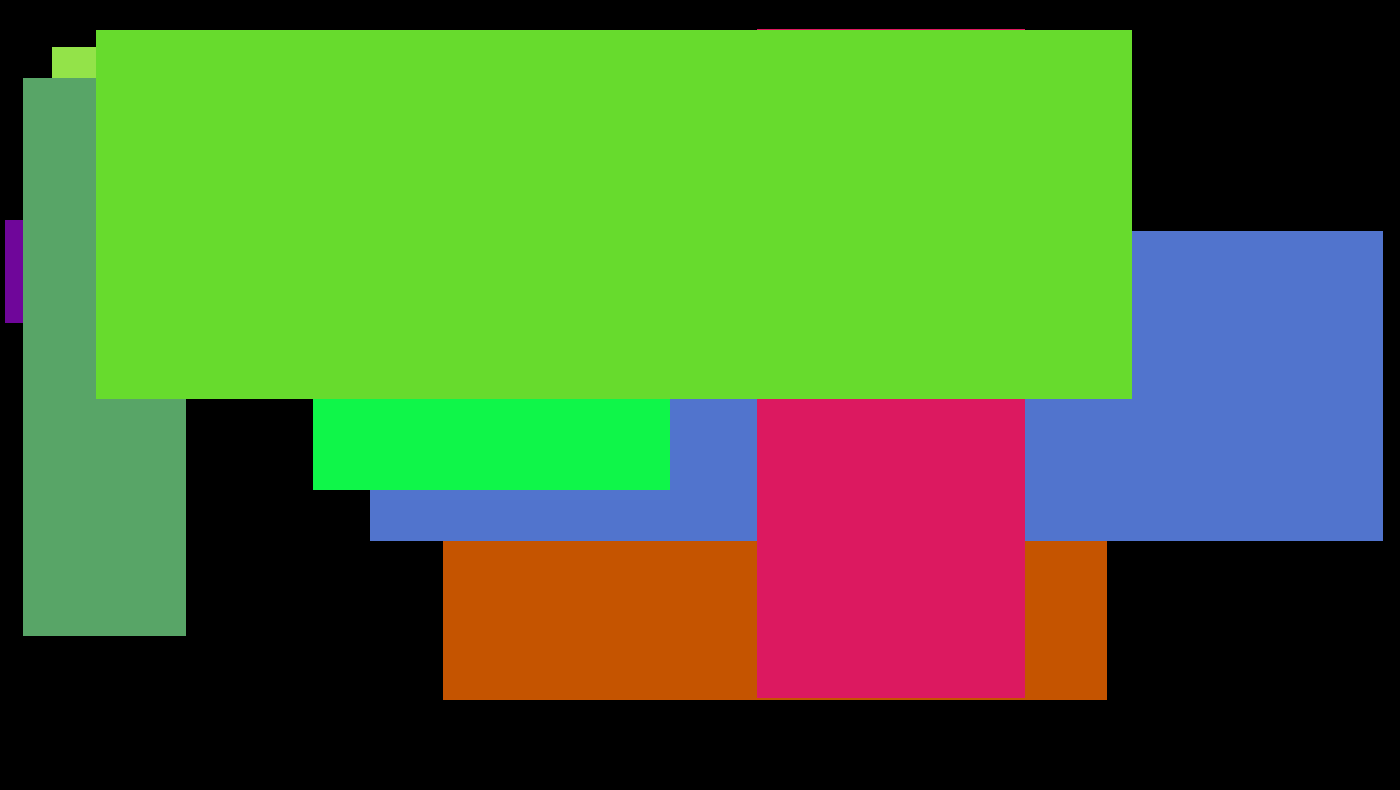### Draw a rounded rectangle

``````for (uint16_t i = 1; i < 30; i++) {
int16_t x0 = rand() % DISPLAY_WIDTH;
int16_t y0 = rand() % DISPLAY_HEIGHT;
int16_t x1 = rand() % DISPLAY_WIDTH;
int16_t y1 = rand() % DISPLAY_HEIGHT;
int16_t r = 10
color_t color = rand() % 0xffff;

hagl_draw_rounded_rectangle(x0, y0, x1, y1, r, color);
}
``````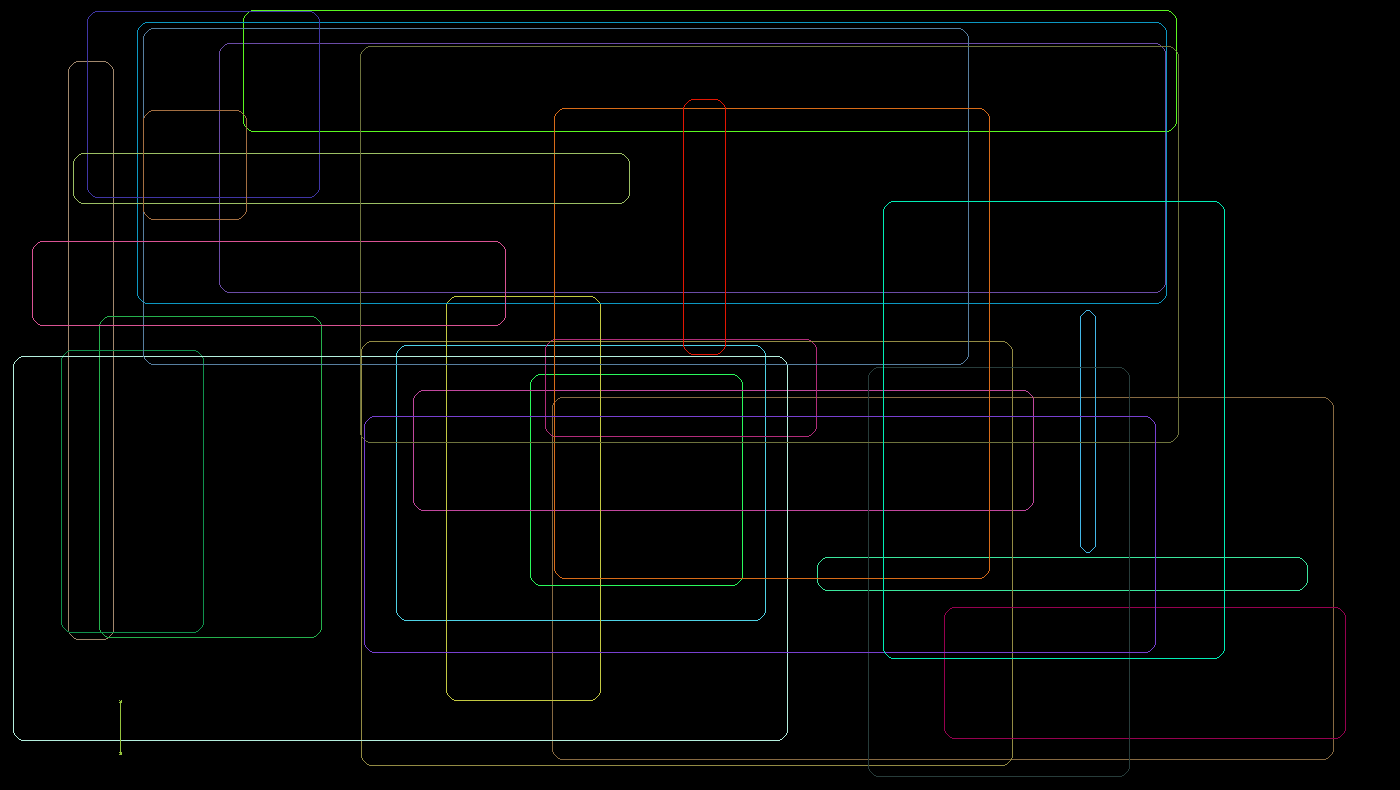### Draw a filled rounded rectangle

``````for (uint16_t i = 1; i < 30; i++) {
int16_t x0 = rand() % DISPLAY_WIDTH;
int16_t y0 = rand() % DISPLAY_HEIGHT;
int16_t x1 = rand() % DISPLAY_WIDTH;
int16_t y1 = rand() % DISPLAY_HEIGHT;
int16_t r = 10
color_t color = rand() % 0xffff;

hagl_fill_rounded_rectangle(x0, y0, x1, y1, r, color);
}
``````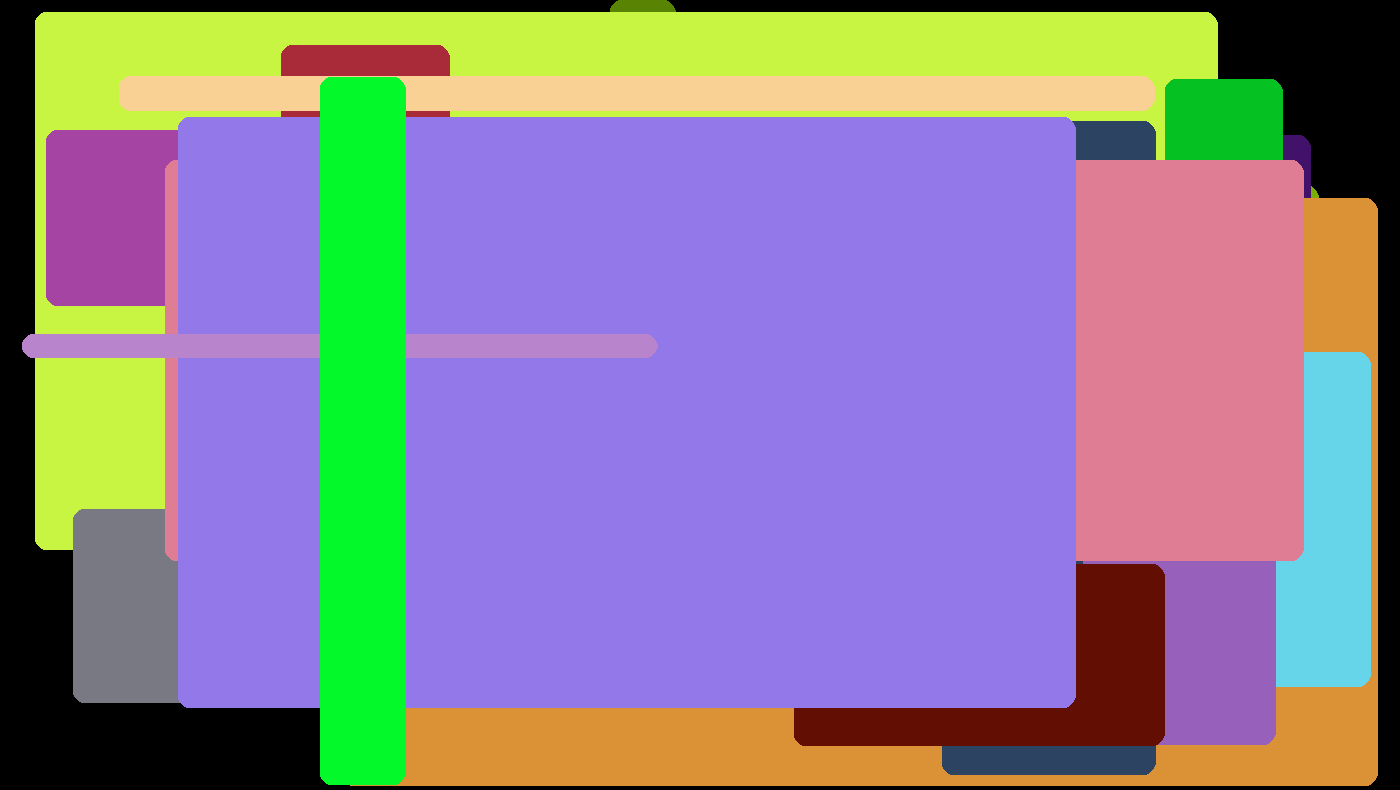### Draw a polygon

You can draw polygons with unlimited number of vertices which are passed as an array. Pass the number of vertices as the first argument.

``````int16_t x0 = rand() % DISPLAY_WIDTH;
int16_t y0 = rand() % DISPLAY_HEIGHT;
int16_t x1 = rand() % DISPLAY_WIDTH;
int16_t y1 = rand() % DISPLAY_HEIGHT;
int16_t x2 = rand() % DISPLAY_WIDTH;
int16_t y2 = rand() % DISPLAY_HEIGHT;
int16_t x3 = rand() % DISPLAY_WIDTH;
int16_t y3 = rand() % DISPLAY_HEIGHT;
int16_t x4 = rand() % DISPLAY_WIDTH;
int16_t y4 = rand() % DISPLAY_HEIGHT;
color_t color = rand() % 0xffff;
int16_t vertices = {x0, y0, x1, y1, x2, y2, x3, y3, x4, y4};

hagl_draw_polygon(5, vertices, color);
``````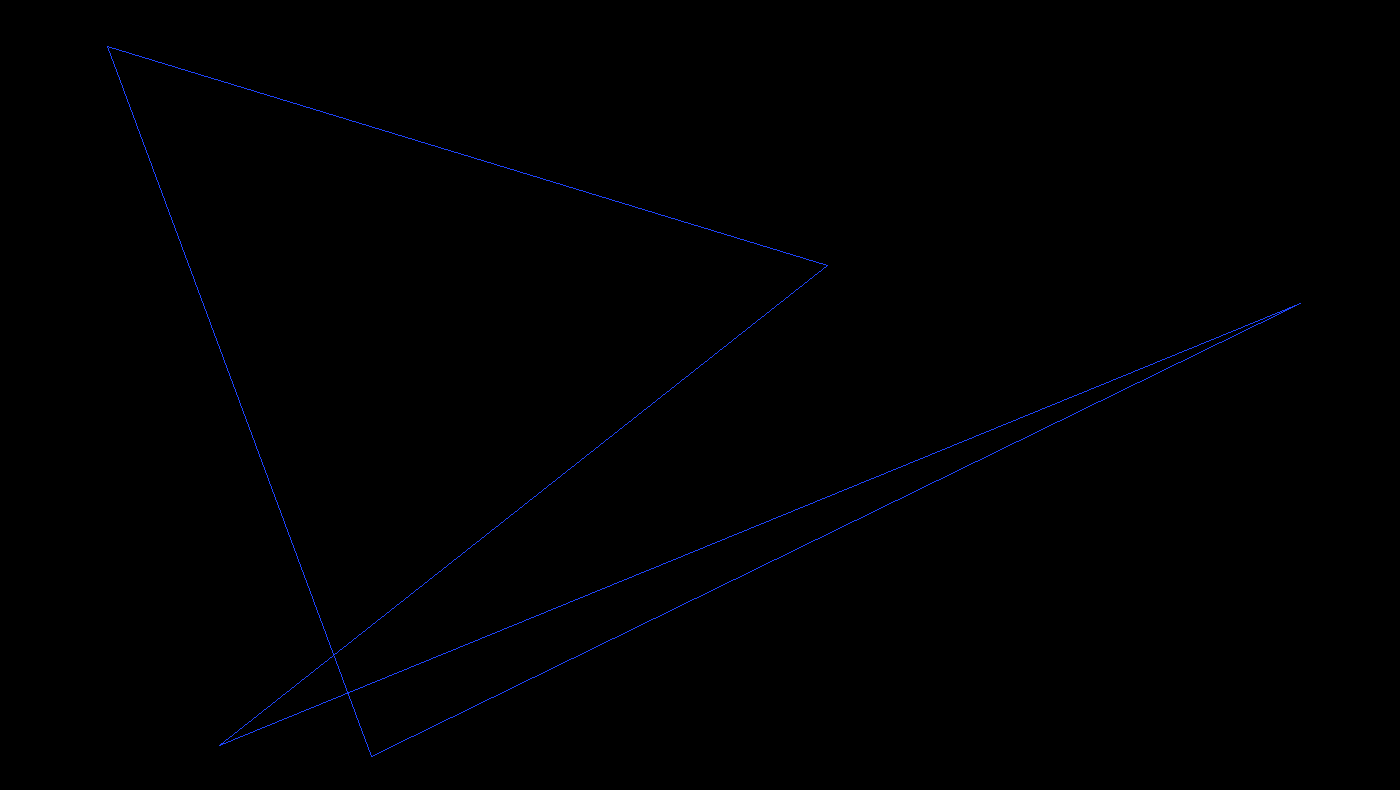### Draw a filled polygon

You can draw filled polygons with up to 64 vertices which are passed as an array. First argument is the number of vertices. Polygon does not have to be concave.

``````int16_t x0 = rand() % DISPLAY_WIDTH;
int16_t y0 = rand() % DISPLAY_HEIGHT;
int16_t x1 = rand() % DISPLAY_WIDTH;
int16_t y1 = rand() % DISPLAY_HEIGHT;
int16_t x2 = rand() % DISPLAY_WIDTH;
int16_t y2 = rand() % DISPLAY_HEIGHT;
int16_t x3 = rand() % DISPLAY_WIDTH;
int16_t y3 = rand() % DISPLAY_HEIGHT;
int16_t x4 = rand() % DISPLAY_WIDTH;
int16_t y4 = rand() % DISPLAY_HEIGHT;
color_t color = rand() % 0xffff;
int16_t vertices = {x0, y0, x1, y1, x2, y2, x3, y3, x4, y4};

hagl_fill_polygon(5, vertices, color);
``````The library supports Unicode fonts in fontx format. It only includes three fonts by default. You can find more at tuupola/fonts repository.

### Put a single char

``````for (uint16_t i = 1; i < 10000; i++) {
int16_t x0 = rand() % DISPLAY_WIDTH;
int16_t y0 = rand() % DISPLAY_HEIGHT;
color_t color = rand() % 0xffff;
char ascii = rand() % 127;

hagl_put_char(ascii, x0, y0, color, font8x8);
}
``````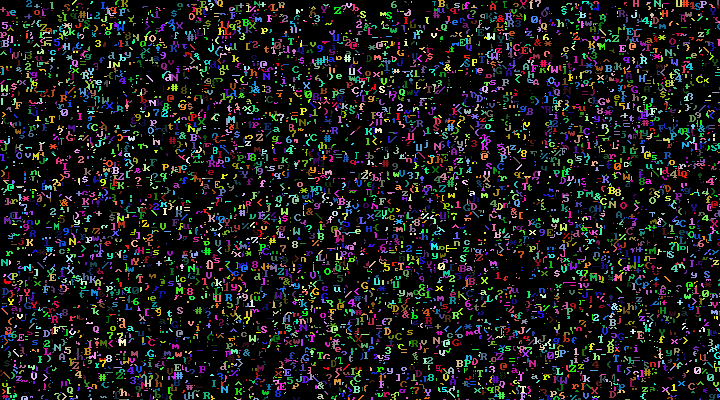### Put a string

The library supports Unicode fonts in fontx format. It only includes three fonts by default. You can find more at tuupola/fonts repository.

``````for (uint16_t i = 1; i < 10000; i++) {
int16_t x0 = rand() % DISPLAY_WIDTH;
int16_t y0 = rand() % DISPLAY_HEIGHT;
color_t color = rand() % 0xffff;

hagl_put_text("YO! MTV raps.", x0, y0, color, font8x8);
}
``````